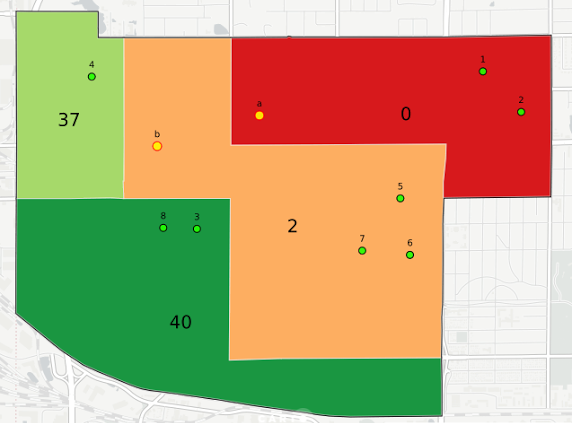Here’s some basic text.

And here’s some italics

Here’s some bold text.

Here’s a bulleted list:

• First item
• second item
• third item

Here’s a numbered list:

1. first
2. second
3. third

Python code block:

``````	import numpy as numpy
def test_function(x,y):
z=np.sum(x,y)
return z
``````

Here is an image: